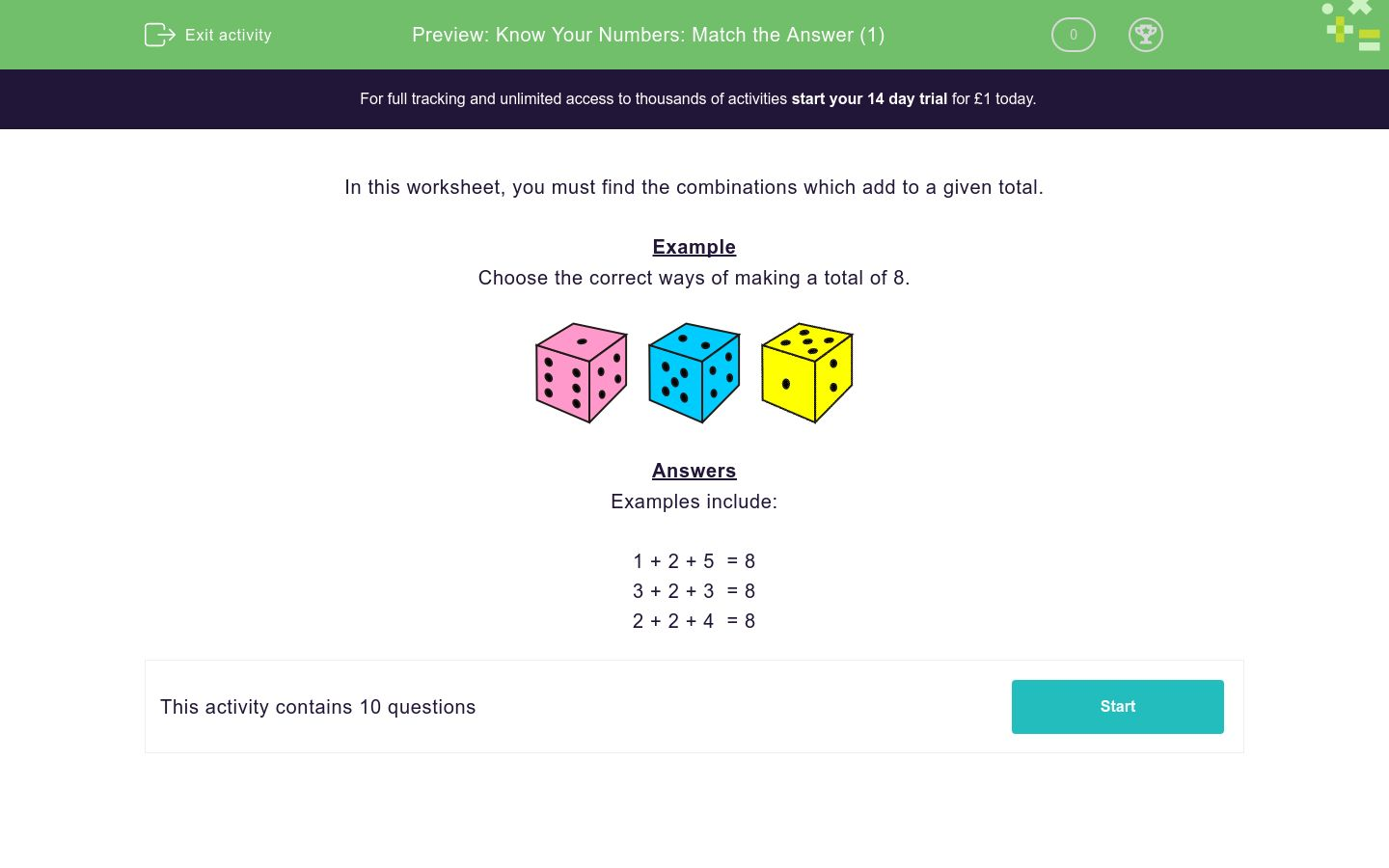### Affordable pricing from £10/month

In this worksheet, students select the correct answer to a series of questions using the addition of three numbers.Key stage:  KS 1

Curriculum topic:   Number: Addition and Subtraction

Curriculum subtopic:   Solve Add/Subtract Problems to 100

Difficulty level:### QUESTION 1 of 10

In this worksheet, you must find the combinations which add to a given total.

Example

Choose the correct ways of making a total of 8.Examples include:

1 + 2 + 5  = 8
3 + 2 + 3  = 8
2 + 2 + 4  = 8

Choose the correct ways of making a total of 8.1 + 2 + 5 = 8

1+2+5

3+2+3

2+3+4

2+2+4

Choose the correct ways of making a total of 9.1 + 3 + 5 = 9

1+2+5

4+4+1

2+3+4

2+2+4

Choose the correct ways of making a total of 10.1 + 3 + 6 = 10

3+2+5

4+4+2

3+3+4

2+2+4

Choose the correct ways of making a total of 12.3 + 3 + 6 = 12

5+4+3

4+4+2

4+4+4

2+2+4

Choose the correct ways of making a total of 8.1 + 2 + 5 = 8

2+4+2

4+4+2

1+3+4

2+2+4

Choose the correct ways of making a total of 10.1 + 4 + 5 = 10

2+4+2

4+4+2

1+3+4

2+2+4

Choose the correct ways of making a total of 11.1 + 5 + 5 = 11

6+4+1

4+4+3

1+3+4

2+2+4

Choose the correct ways of making a total of 13.3 + 5 + 5 = 13

6+4+1

4+4+3

6+3+4

6+2+5

Choose the correct ways of making a total of 14.4 + 5 + 5 = 14

6+4+1

4+4+3

6+4+4

6+2+5

Choose the correct ways of making a total of 15.4 + 5 + 6 = 15

6+4+1

3+6+6

6+4+4

6+4+5

• Question 1

Choose the correct ways of making a total of 8.1 + 2 + 5 = 8

1+2+5
3+2+3
2+2+4
EDDIE SAYS

A = 1+2+5 = 8
B = 3+2+3 = 8
C = 2+2+4 = 8

• Question 2

Choose the correct ways of making a total of 9.1 + 3 + 5 = 9

4+4+1
2+3+4
EDDIE SAYS

2+3+4 = 9
4+4+1 = 9

• Question 3

Choose the correct ways of making a total of 10.1 + 3 + 6 = 10

3+2+5
4+4+2
3+3+4
EDDIE SAYS

3+2+5 = 10
4+4+2 = 10
3+3+4 = 10

• Question 4

Choose the correct ways of making a total of 12.3 + 3 + 6 = 12

5+4+3
4+4+4
EDDIE SAYS

4+4+4 = 12
5+4+3 = 12

• Question 5

Choose the correct ways of making a total of 8.1 + 2 + 5 = 8

2+4+2
1+3+4
2+2+4
EDDIE SAYS

2+4+2 = 8
1+3+4 = 8
2+2+4 = 8

• Question 6

Choose the correct ways of making a total of 10.1 + 4 + 5 = 10

4+4+2
EDDIE SAYS

4+4+2 = 8

• Question 7

Choose the correct ways of making a total of 11.1 + 5 + 5 = 11

6+4+1
4+4+3
EDDIE SAYS

4+4+3 = 8
6+4+1 = 8

• Question 8

Choose the correct ways of making a total of 13.3 + 5 + 5 = 13

6+3+4
6+2+5
EDDIE SAYS

6+4+3 = 13
6+2+5 = 13

• Question 9

Choose the correct ways of making a total of 14.4 + 5 + 5 = 14

6+4+4
EDDIE SAYS

6+4+4 = 13

• Question 10

Choose the correct ways of making a total of 15.4 + 5 + 6 = 15

3+6+6
6+4+5
EDDIE SAYS

6+4+5 = 15
3+6+6 = 15

---- OR ----

Sign up for a £1 trial so you can track and measure your child's progress on this activity.

### What is EdPlace?

We're your National Curriculum aligned online education content provider helping each child succeed in English, maths and science from year 1 to GCSE. With an EdPlace account you’ll be able to track and measure progress, helping each child achieve their best. We build confidence and attainment by personalising each child’s learning at a level that suits them.

Get started